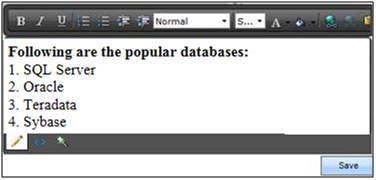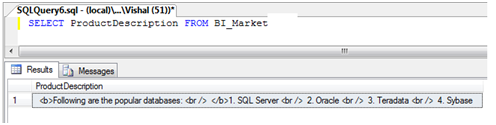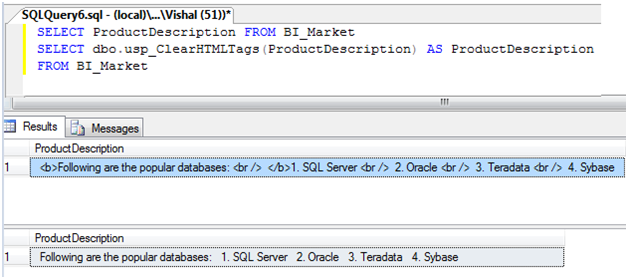# Migrate SQLite to MySQL

I’m developing a huge app made with Xamarin Forms in my company. A problem was to create all tables in the device with SQLite to the main database MySql.I found a way to create all tables quickly with a tool called SQLite to MySQL.

SQLite-to-MySQL is a powerful and reliable tool to convert SQLite databases to MySQL, MariaDB or Percona format. The program has high performance due to direct connection to source and destination databases (it does not use ODBC or any other middleware software). Command line support allows to script, automate and schedule the conversion process.Features

• All versions of Linux/Unix and Windows MySQL servers are supported
• Option to customize resulting table structure
• Convert indexes and relationships between tables
• Stores conversion settings into profile
• Option to filter data using SELECT-queries
• Option to merge SQLite data into an existing MySQL database
• Option to synchronize MySQL database with SQLite data
• Support for multibyte character sets
• Option to export SQLite database into MySQL script file
• Verified compatibility with MariaDB and Percona
• Option to customize MySQL storage engine and MySQL charset
• Special approach for Virtual Server users
• Command line support
• Easy-to-use wizard-style interface
• Full install/uninstall support

# How to Convert an IP Address to IP Number in SQL

The process of converting an IP Address to an IP Number is relatively simple. For example take the IP Address 192.168.1.0 - To convert it to an IP Number you would do the following.

Number = (256*256*256*192) + (256*256*168) + (256*1) + (0)

Unfortunately MS SQL doesn't provide any native Split functionality to split the decimal separated ip address into the small pieces of data that we could easily work with. We thought we were going to have to do some nasty string dissection using Substring and CharIndex and then we stumbled upon the beautiful Parsename function. Apparently this function was originally designed to help developers navigate through the MS SQL naming structure (ie databasename.dbo.tablename). For our purposes it worked great to pull apart our decimal separated string so we could perform calculations on each piece of data.

```update IPTable set IPNumber =
256 * 256 * 256 * CAST(PARSENAME(@ipaddress, 4) AS float) +
256 * 256 * CAST(PARSENAME(@ipaddress, 3) AS float) +
256 * CAST(PARSENAME(@ipaddress, 2) AS float) +

Happy coding!

# How to use REPLACE() within NTEXT columns in SQL Server

SQL has an incredibly useful function, `REPLACE()`, which replaces all occurrences of a specified string with another string, returning a new string. It works great with all forms of `NCHAR` and `NVARCHAR` fields. It does not, however, work with `NTEXT` fields.

Fear not — there’s an easy workaround, thanks to type-casting and SQL 2005’s `NVARCHAR(max)` datatype. Here’s the process in an nutshell.

1. Cast the `NTEXT` field to the `NVARCHAR(max)` datatype using the `CAST` function.
2. Perform your `REPLACE` on the output of #1.
3. Cast the output of #2 back to `NTEXT`. (Not really required, but it does get us back to where we started.

A simple SQL query illustrates this.

```select cast(replace(cast(myntext as nvarchar(max)),'find','replace') as ntext)
from myntexttable```

If you’re using SQL 2000, you’re out of luck, as `NVARCHAR(max)` first appeared in SQL 2005. However, if your `NTEXT` field is less than 8000 characters, you can cast it to `VARCHAR(8000)` — the largest possible `VARCHAR` size — to accomplish the same.

[Note #1: This solution below will also work with `TEXT` fields. Simply replace `NTEXT` with `TEXT`, and `NVARCHAR` with `VARCHAR`.]

[Note #2: `NTEXT` fields are depreciated in SQL 2005 in favor of `NVARCHAR(max)`, so avoid using `NTEXT` and you’ll avoid this problem altogether in the future.]

# How can I know when SQL Full Text Index Population is finished?When you execute some queries on your SQL Server, are you sure the catalog is being imported?

With this simple script you can know which is the Index status.

```DECLARE @CatalogName VARCHAR(MAX)
SET     @CatalogName = 'TEST_FullIndex'

SELECT FULLTEXTCATALOGPROPERTY(@CatalogName,'ItemCount') as NumberOfItems,
FULLTEXTCATALOGPROPERTY(@CatalogName,'ImportStatus') as ImportStatus,
'PopulateCompletionAge'), '1/1/1990') AS LastPopulated,
(SELECT CASE FULLTEXTCATALOGPROPERTY(@CatalogName,'PopulateStatus')
WHEN 0 THEN 'Idle'
WHEN 1 THEN 'Full Population In Progress'
WHEN 2 THEN 'Paused'
WHEN 3 THEN 'Throttled'
WHEN 4 THEN 'Recovering'
WHEN 5 THEN 'Shutdown'
WHEN 6 THEN 'Incremental Population In Progress'
WHEN 7 THEN 'Building Index'
WHEN 8 THEN 'Disk Full.  Paused'
WHEN 9 THEN 'Change Tracking' END) AS Status
FROM sys.fulltext_catalogs AS cat```

# How to remove HTML tags from data with SQL

The purpose of this article is to provide a way of cleaning up of HTML tags within the data. When we use various styles or tabular format data in UI using Rich Text Editor/ Rad Grid etc, it will save data in database with HTML tags.When you save in database this kind of field you have:An HTML element starts with a start tag (<p>) and ends with end tag (<p/>) and everything between Start tag and End tag is HTML element. e.g.

<b>Following are the popular databases: <br /> </b>1. SQL Server <br /> 2. Oracle <br /> 3.

We are using SQL Server CHARINDEX function to get the positions of ‘<’ and ‘>’ and once found replacing the string between <….> with blank using STUFF function. We are using WHILE Loop that will run till presence of ‘<’ & ‘>’ in the string. Below is the UDF script that performs HTML Tags clean up from data.

```SET ANSI_NULLS ON
GO

SET QUOTED_IDENTIFIER ON
GO

CREATE FUNCTION [dbo].[usp_ClearHTMLTags]
(@String NVARCHAR(MAX))
RETURNS NVARCHAR(MAX)

AS
BEGIN
DECLARE @Start INT,
@End INT,
@Length INT

WHILE CHARINDEX('<', @String) > 0 AND CHARINDEX('>', @String, CHARINDEX('<', @String)) > 0
BEGIN
SELECT  @Start  = CHARINDEX('<', @String),
@End    = CHARINDEX('>', @String, CHARINDEX('<', @String))
SELECT @Length = (@End - @Start) + 1

IF @Length > 0
BEGIN
SELECT @String = STUFF(@String, @Start, @Length, '')
END
END
RETURN @String
END
GO```

By using above UDF, We can clean the HTML tags from the data.# SQL SERVER – Find Current Location of Data and Log File of All the Database

As I am doing lots of experiments on my SQL Server test box, I sometime gets too many files in SQL Server data installation folder – the place where I have all the .mdf and .ldf files are stored. I often go to that folder and clean up all unnecessary files I have left there taking up my hard drive space.

I run following query to find out which .mdf and .ldf files are used and delete all other files. If your SQL Server is up and running OS will not let you delete .mdf and .ldf files any way giving you error that file already in use. This list also helps sometime to do documentation of which files are in being used by which database.

```SELECT name, physical_name AS current_file_location
FROM sys.master_files
```

Following is the output of files used by my SQL Server instance.# How to truncate log file in SQL Server

In SQL Server data is stored using two physical files:

1. (.mdf) extension which contains the data.
2. (.ldf) extension which contains log.

Log file size increases very rapidly and depend on the operation performed on the data. After a long time period this file becomes too large. When log file is too large it takes time to perform some operations like (attach, de-attach, backup, restore... etc ).

Step 1. Copy/type the below given SQL.

Step 2. Change @DBName to <Database Name>, @DBName_log to <Log File Name>

Step 3. Execute the SQL.

```ALTER DATABASE @DBName SET RECOVERY SIMPLE WITH NO_WAIT
DBCC SHRINKFILE(@DBName_log, 1)
ALTER DATABASE @DBName SET RECOVERY FULL WITH NO_WAIT
GO  ```

# PSC.Search: a new implementation of Levenshtein distance algorithm

This article describes a simple implementation of the string search. It can be used for approximate string matching (for more information, see http://en.wikipedia.org/wiki/Fuzzy_string_searching).

Other algorithms for approximate string searching exist (e.g., Soundex), but those aren't as easy to implement. The algorithm in this article is easy to implement, and can be used for tasks where approximate string searching is used in an easy way.

The algorithm used the Levenshtein-distance for determining how exact a string from a word list matches the word to be found. Information about the Levenshtein-distance can be found at http://en.wikipedia.org/wiki/Levenshtein_distance.

## C# implementation

```using System;
using System.Collections.Generic;
using System.Linq;
using System.Text;

namespace PSC.Search.Demo
{
class Program
{
static void Main(string[] args)
{
string word = "Pure Source Code";
List<string> wordList = new List<string>
{
"Code Project",
"Pure SourceCOde",
"sourcecode",
"puresourcecode",
"Source Kode",
"Kode Project",
"Other Source"
};

List<wordrank> foundWords =
FuzzySearch.Search(word, wordList, 0.30);

foundWords.ForEach(i => Console.WriteLine(i.Word +
" (" + i.Rank.ToString() +
")"));
}
}
}

```

Output:A basic approach is shown. Instead of the Levenshtein-distance, a more optimized algorithm could be used - but here, a quite simple implementation is given for clarity reasons.

```public static int LevenshteinDistance(string src, string dest)
{
int[,] d = new int[src.Length + 1, dest.Length + 1];
int i, j, cost;
char[] str1 = src.ToCharArray();
char[] str2 = dest.ToCharArray();

for (i = 0; i <= str1.Length; i++)
{
d[i, 0] = i;
}

for (j = 0; j <= str2.Length; j++)
{
d[0, j] = j;
}

for (i = 1; i <= str1.Length; i++)
{
for (j = 1; j <= str2.Length; j++)
{
if (str1[i - 1] == str2[j - 1])
cost = 0;
else
cost = 1;

d[i, j] =
Math.Min(
d[i - 1, j] + 1,                    // Deletion
Math.Min(
d[i, j - 1] + 1,                // Insertion
d[i - 1, j - 1] + cost));       // Substitution

if ((i > 1) && (j > 1) &&
(str1[i - 1] == str2[j - 2]) &&
(str1[i - 2] == str2[j - 1]))
{
d[i, j] = Math.Min(d[i, j], d[i - 2, j - 2] + cost);
}
}
}

return d[str1.Length, str2.Length];
}

```

In the search process, for each word in the wordlist, the Levenshtein-distance is computed, and with this distance, a score. This score represents how good the strings match. The input argument fuzzyness determines how much the strings can differ.

```public static List<wordrank> Search(string word, List wordList,
double fuzzyness)
{
List<wordrank> foundWords = new List<wordrank>();

foreach (string s in wordList)
{
// Calculate the Levenshtein-distance:
int levenshteinDistance = LevenshteinDistance(word, s);

// Length of the longer string:
int length = Math.Max(word.Length, s.Length);

// Calculate the score:
double score = 1.0 - (double)levenshteinDistance / length;

// Match?
if (score > fuzzyness)
foundWords.Add(new WordRank() { Rank = score, Word = s });
}

return foundWords;
}```

## SQL implementation

Also I've implemented a version for SQL.

```CREATE FUNCTION edit_distance(@s1 nvarchar(3999), @s2 nvarchar(3999))
RETURNS int
AS
BEGIN
DECLARE @s1_len int, @s2_len int
DECLARE @i int, @j int, @s1_char nchar, @c int, @c_temp int
DECLARE @cv0 varbinary(8000), @cv1 varbinary(8000)

SELECT
@s1_len = LEN(@s1),
@s2_len = LEN(@s2),
@cv1 = 0x0000,
@j = 1, @i = 1, @c = 0

WHILE @j <= @s2_len
SELECT @cv1 = @cv1 + CAST(@j AS binary(2)), @j = @j + 1

WHILE @i <= @s1_len
BEGIN
SELECT
@s1_char = SUBSTRING(@s1, @i, 1),
@c = @i,
@cv0 = CAST(@i AS binary(2)),
@j = 1

WHILE @j <= @s2_len
BEGIN
SET @c = @c + 1
SET @c_temp = CAST(SUBSTRING(@cv1, @j+@j-1, 2) AS int) +
CASE WHEN @s1_char = SUBSTRING(@s2, @j, 1) THEN 0 ELSE 1 END
IF @c > @c_temp SET @c = @c_temp
SET @c_temp = CAST(SUBSTRING(@cv1, @j+@j+1, 2) AS int)+1
IF @c > @c_temp SET @c = @c_temp
SELECT @cv0 = @cv0 + CAST(@c AS binary(2)), @j = @j + 1
END

SELECT @cv1 = @cv0, @i = @i + 1
END

RETURN @c
END```

Usage:

```select
dbo.edit_distance('Fuzzy String Match','fuzzy string match'),
dbo.edit_distance('fuzzy','fuzy'),
dbo.edit_distance('Fuzzy String Match','fuzy string match'),
dbo.edit_distance('levenshtein distance sql','levenshtein sql server'),
dbo.edit_distance('distance','server')```

Happy coding!